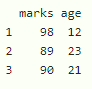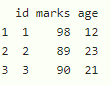# Select Only Numeric Columns from DataFrame in R

In this article, we will discuss how to select only numeric columns from dataframe in R Programming Language.

## Method 1: Using Dplyr package

We can use select_if() function to get numeric columns by calling the function with the dataframe name and isnumeric() function that will check for numeric columns.

Syntax:

select_if(dataframe, is.numeric)

where,

• dataframe is the input dataframe
• is.numeric is used to get the numeric columns

Example: R program to get numeric columns from dataframe using dplyr

## R

 `# load the package dplyr` `library``(``"dplyr"``)`   `# create  a dataframe with 4 columns and 3 rows` `data=``data.frame``(``"webtechnologies"``=``c``(``"php"``,``"html"``,``"js"``), ` `                ``marks=``c``(98,89,90),` `                ``age=``c``(12,23,21),` `                ``name=``c``(``"bobby"``,``"ojaswi"``,``"ramya"``))`   `    `  `# get numeric columns using dplyr() function             ` `print``(``select_if``(data, is.numeric))`

Output:Example: R program to get numeric columns from dataframe using dplyr

## R

 `# load the package dplyr` `library``(``"dplyr"``)`   `# create  a dataframe with 4 columns and 3 rows` `data=``data.frame``(id=``c``(1,2,3), ` `                ``marks=``c``(98,89,90),` `                ``age=``c``(12,23,21),` `                ``name=``c``(``"bobby"``,``"ojaswi"``,``"ramya"``))`   `    `  `# get numeric columns using dplyr() function             ` `print``(``select_if``(data, is.numeric))`

Output:## Method 2: Using lapply() function

we will use lapply() function to get the numeric columns. Here, lapply() function is called with unlist() and the dataframe name and isnumeric() function are passed to it as parameters.

Syntax:

unlist(lapply(dataframe, is.numeric))

where,

• dataframe is the input dataframe
• is.numeric is used to check each dataframe column is numeric or not
• unlist function is used to unlist the dataframe

Finally, pass this lapply() to the dataframe index

Syntax:

dataframe[,unlist(lapply(data, is.numeric))]

Where, dataframe is the input dataframe

Example: R program to get numeric columns from dataframe using base R

## R

 `# create  a dataframe with 4 columns and 3 rows` `data=``data.frame``(id=``c``(1,2,3), ` `                ``marks=``c``(98,89,90),` `                ``age=``c``(12,23,21),` `                ``name=``c``(``"bobby"``,``"ojaswi"``,``"ramya"``))`   `    `  `# get numeric columns using dplyr() function             ` `print``(data[,``unlist``(``lapply``(data, is.numeric))])`

Output:Whether you're preparing for your first job interview or aiming to upskill in this ever-evolving tech landscape, GeeksforGeeks Courses are your key to success. We provide top-quality content at affordable prices, all geared towards accelerating your growth in a time-bound manner. Join the millions we've already empowered, and we're here to do the same for you. Don't miss out - check it out now!

Previous
Next